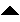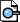Next: Lectures Up: Introduction to the Bootstrap: Previous: Logistics   Index

Subsections

# Labs

## Lab 1, April 8th, 2002: to hand in before April 11th

Computing Lab Section #1

1. Setting Things Up.

Make a course directory.
    elaine20:~> mkdir s208
elaine20:~> cd s208

Make a file called standnorm.m with the following lines:
    function out=standnorm(n)
out=randn(1,n);


If you are working on a leland machine, you could also simply copy the file from the course directory:

     cp /usr/class/stat208/lab/standnorm.m .

2. Starting Matlab. Matlab is installed on all leland machines - elaine, tree, etc.
Just type:
    elaine20:~> matlab

You should see the matlab prompt:
    >>


3. Warming Up. We will use the standnorm function to generate a vector of 15 random numbers.
    >> standnorm(15)

ans =

Columns 1 through 5

-0.4326   -1.6656    0.1253    0.2877   -1.1465

Columns 6 through 10

1.1909    1.1892   -0.0376    0.3273    0.1746

Columns 10 through 15

-0.1867    0.7258   -0.5883    2.1832   -0.1364


Question 1   Did you get the same answer as I did? Many of you will. The random number generator is not really random! How could you explain this?

4. Distribution of Sample Mean

Try typing in: (2)
    >> sampling=mean(standnorm(15))

sampling =

0.2158

sampling is the mean of a sample of size 15.

Next, (1)

    >> sampling=[sampling mean(standnorm(15))]

sampling =

0.2158    -0.1201

What does this command do?

Now repeat the previous command 10 more times. Stop when you see sampling is a vector of length 12. (hint: yourkey may save you a lot of typing in matlab).

Question 2   If the original random variables come from the standard Normal distribution, N(0,1), what is the variance of the means of samples of size 15?

5. Distribution of Sample Median

How could we get the mean and variance of the medians of 100 samples of size 15?

1. A clumsy approach. Use a for loop to repeat commands in (1).

If we want to discard the sampling vector we have so far, we could do:

    >> sampling=zeros(0);
>> for i=1:100
sampling=[sampling median(standnorm(15))];
end


Question 3   From a computational point of view, this is lousy. Why?

2. A much cleaner for loop.
>> sampling=zeros(100,1);
>> for i=1:100
sampling(i)=median(standnorm(15));
end


3. Is the loop in (b) actually faster than the one in (a)?
Try timing it with
1. cputime - cpu times;
hint

>> start=cputime;
...
>> ending = cputime-start;


2. etime - elapsed time;
3. tic toc - stopwatch timer;
4. flops - number of floating point operation count;

hint: Use the help command in Matlab.

Question 4   Compare time used to complete loops in (a) and (b) with above commands. Do the two loops run equally fast? Explain why, or why not.

Question 5   Using the results from 5(b), how would you estimate the mean and the variance of the medians of samples of size 15?

6. Confidence Interval, Quantiles, etc....

Question 6   Using the results from 5(b), how do you find the 95% confidence interval for the sample median? How do you find the quantiles of the sample median?

You can download a pdf file of this lab here.

## Lab 2, April 17th, 2002, class time:11.00am: to hand in before April 20th

1. Setting Things Up.
Use your course directory, by typing: elaine20: > cd s208.

Make a file called myrand.m with the following lines:

function out=myrand(m,n,range)
%Returns a vector of draws
%of integers from 1 to n,
%with replacement
out=floor(rand(m,n)*range)+1;


Make a file called bsample.m with the following lines:

function out=bsample(orig)
%Function to create one resample from
%the original sample orig, where
%orig is the original data, and is a
%matrix with nrow observations and ncol variables
[n,p]=size(orig);
indices=myrand(1,n,n);
out=orig(indices,:);


Make a file containing the complete 82 point law school data by downloading it from the class web directory, http://www.stanford.edu/class/stats208/law82. You can use save as in the netscape file option. Or you can copy the file law82 from the /usr/class/stats208/lab/ directory.

Start Matlab. Matlab is installed on all leland machines - elaine, tree, etc.
You should see the matlab prompt: » Then type format compact to get more on your screen.

2. Using the helpdesk to find some functions for which you don't know the exact name. Type helpdesk at the matlab prompt and, in the new help'' window, go to search'' to find the name of the function that calculates the correlation coefficient.

Question 7   What's the name of the function that calculates the correlation coefficient? At the matlab prompt, try typing help followed by the name of this function and then type followed by the name of this function and briefly explain what each of the two functions help and type does. Type lookfor correlation and explain what the function lookfor does.

3. Using some of the GUI stat tools: disttool and randtool.

Using the randtool, generate two poisson data of size 1000, one with parameter, one with parameter. To do that type randtool at the matlab prompt. Adjust the name of the distribution, the parameter value and the size of the dataset. To save data you generated using randtool, click on Output. Save data sets as Poisson1 and Poisson2. Return to Matlab and answer the following questions:

Question 8   What is the estimate of the standard error of the difference in Poisson means?

Question 9   What is your estimate of the variance of the difference in Poisson means? Compare this estimate to the theoretical variance of the difference in Poisson means.

Question 10   Make a histogram of the difference in the two Poisson random variables.

4. Working with law82 data.

Question 11   Make a scatterplot of law82 dataset. To do that, you have to load the data into MATLAB by typing:
    load law82


Question 12   Write a modification to the function bsample that takes two arguments orig and k and creates one subsample of size k from orig. Call this function bsamplesizek and store it in a file called bsamplesizek.m

Use your function to draw 1000 subsamples of size 15 from law82 dataset.

Question 13   Compute correlation coefficients for each of the subsamples and create a histogram.

You can download a pdf file of this lab here.

# Homeworks

## Homework 1, Due April 17th, 2002: to hand in before lab

From the text:
Page 15, 2.4.
Page 28-30 : 3.1, 3.5, 3.10.

Hints for Efron & Tibshirani, Problem 2.4
We want to calculatemedian is equal to a certain. We do this by calculating the difference between probabilities of getting a median that is greater than or equal toand getting a median that is greater than or equal to. That is,. To find1. Think what is the minimum requirements'' forsuch that its median is larger than a number.
2. Calculate the probability in (1). How many ways you could get a bootstrap sample that fulfill the minmum requirements'' This should be## Homework 2:due Friday April, 26th

Read Chapter 11 of text, do exercices 10.8, 11.8, 11.12, 11.13.Next: Lectures Up: Introduction to the Bootstrap: Previous: Logistics   Index
Susan Holmes 2002-04-25